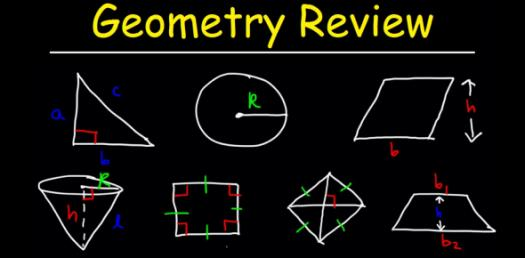# Geometric Test: Can You Pass This Quiz?

10 Questions | Total Attempts: 61SettingsThis quiz will test your ability to find the rule for the geometric sequence, and the common ratio when you are given the first three terms. Conventions: numbers are to a maximum of 2 decimal places. If two numbers are requested, they are separated by a comma only - no spaces. Rules contain no spaces.

• 1.
What type of sequence is formed by the terms above?
• A.

Arithmetic

• B.

Geometric

• C.

Neither Arithmetic or Geometric

• 2.
What are the next three terms of the sequence above?
• 3.
A geometric rule has the form : Given the sequence above, what are the values for the rule above (in order) ?
• 4.

• 5.

• 6.
What is the common ratio for the sequence above?
• 7.
What is the common ratio for the sequence above?
• 8.
What is the common ratio for the sequence above?
• 9.
What is the common ratio for the sequence above?
• 10.
What is the common ratio for the sequence above?
• 11.
What is the common ratio for the sequence above?
• 12.
What is the common ratio for the sequence above?
• 13.
What is the common ratio for the sequence above?
• 14.
What is the common ratio for the sequence above?
• 15.
What is the common ratio for the sequence above?
• 16.
What is the common ratio for the sequence above?
• 17.
What is the common ratio for the sequence above?
• 18.
Above is a geometric series. The value given for 'n' is the number of values in this series. What is the total value of this finite geometric series?
• 19.
What type of sequence is formed by the terms above?
• A.

Arithmetic

• B.

Geometric

• C.

Neither Arithmetic or Geometric

• 20.
What type of sequence is formed by the terms above?
• A.

Arithmetic

• B.

Geometric

• C.

Neither Arithmetic or Geometric

• 21.
What type of sequence is formed by the terms above?
• A.

Arithmetic

• B.

Geometric

• C.

Neither Arithmetic or Geometric

• 22.
What type of sequence is formed by the terms above?
• A.

Arithmetic

• B.

Geometric

• C.

Neither Arithmetic or Geometric

• 23.
What type of sequence is formed by the terms above?
• A.

Arithmetic

• B.

Geometric

• C.

Neither Arithmetic or Geometric

• 24.
What type of sequence is formed by the terms above?
• A.

Arithmetic

• B.

Geometric

• C.

Neither Arithmetic or Geometric

• 25.
What type of sequence is formed by the terms above?
• A.

Arithmetic

• B.

Geometric

• C.

Neither Arithmetic or Geometric

Related TopicsBack to top